# How To Get A Perfect 36 On The ACT MathIn this post, I will show you the topics that you need to know, tell you the strategies you need to use, and give you the formulas that you will need to memorize to get a perfect 36 on the Math. Even if you do not think you can get a perfect 36, this article will help every student increase his or her ACT Math score by 2-3 points.

On more recent ACTs, the ACT Math section has begun to include some less commonly known topics that many students have not covered in the classroom. These topics are not especially difficult, but, for students who have learned the rules, problems on these topics are nearly impossible.

In this post, I will be focusing on these higher-level topics that you are less likely to be familiar with. I will introduce you to the topic, cover how it works, and show you how the problems may appear on the ACT. All formulas that you should memorize for test day will be in red.

Topics covered in this post are:

• Trapezoids

• Circles

• Ellipses

• Law of Sines and Law of Cosines

• Vertical and Horizontal Asymptotes

• Complex Numbers and the Complex Number Plane

• Matrices

Trapezoids

Trapezoid problems are usually very easy to solve as long as you know the formula to solve for the area of a trapezoid. However, many students do not know the trapezoid formula!As long as you memorize the area formula, trapezoid questions should be pretty simple.

Practice:

In the trapezoid ABCD below, line AB is 10 inches long, line DC is 15 inches long, and line EF is 8 inches long. What is the area of the trapezoid in square inches?Scroll down for the answer and explanation.

Using the formula and the information given in the problem,

1/2 (10 + 15) x 8 = 100.

Circles

As long as you memorize the circle formula and understand how it works, you will be prepared for all of the circle questions on the ACT.

For a circle with center (h,k) and radius r: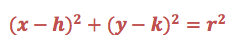Practice: Write the equation for a circle with center (-3, 5) and a radius of 8.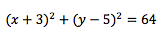Ellipses

Ellipses often appear towards the end of the ACT Math Test. For these problems, you will need to memorize the ellipse formula and know how an ellipse appears on the coordinate plane.For an ellipse with center (h,k):Instead of having a radius, ellipses have a major and minor axis. You can think of the "a" value as the radius along the x-axis, and you can think of the "b" value as the radius along the y-axis.

Here's an example of an ellipse equation with some numbers inserted, so you can see how it appear on a graph.Here is an example of how an ellipse problem might appear on the ACT.

Which of the following is the correct equation for the ellipse seen below?Scroll down for the answer and solution.

The center of the ellipse is at the origin, so the values for h and k are both zero. Since the distance from the center to the edge of the ellipse in the horizontal direction is 5, the value of a is 5. Similarly, the distance from the center of the ellipse to the edge in the vertical direction is 4, so the value of b is 4. If we plug these values into the standard ellipse equation, we get the equation found in answer choice C.

Beyond the basics of SOH-CAH-TOA, you will also need to know some other advanced trigonometry topics. We will not cover the basic SOH-CAH-TOA here or the basics of inverse trigonometric functions. However, those will be on the ACT, so make sure that you know how to use them!Angles in trigonometry can be expressed in radians or degrees. On the ACT, you will need to be able to switch between radians and degrees.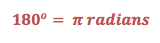Just use this conversion factor to switch between degrees and radians.

For example, if I needed to turn 540 degrees into radians, I would setup the problem like so:If I instead need to turn (7pi)/4 radians into degrees, I would setup the problem like so:Practice:

1) Convert 135 degrees into radians.

2) Convert (8pi)/3 radians into degrees.

2) 480 degrees

Graphing Sine and Cosine

On the ACT, you may need to recognize a sine and cosine graph.

y = sin(x) y = cos(x)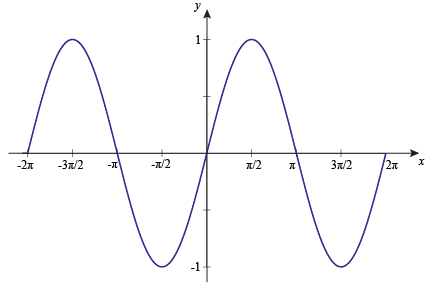The big difference to notice here is that the sin(x) graph intersects the origin while the cos(x) graph intersects the y-axis at y=1.

On the ACT, you will to be able to recognize the graphs of these functions and also have to be familiar with how these graphs shift and change.• A is the amplitude of the function (half of the total height of the graph)

• (2pi/B) is the period for a sine and cosine function. (pi/B) is the period for a tangent function. The period of a function is the horizontal length of the repeating portion.

• C shifts the graph horizontally. A positive value of C shifts the graph left while a negative value of C shifts the graph right.

• D shifts the graph vertically. A positive value of D shifts the graph up while a negative value of D shifts the graph down.

Finding the Amplitude and Period of a Trigonometric Function

Finding the amplitude and period for a trig function is easy once you know where to look. The amplitude is simply the value of A as seen above. The period is a bit more tricky, but just remember the value of B is whatever the x in the function is multiplied by. Let's work through a few examples.

Find the period and amplitude for the following functions.

y = 6 cos (4x) + 3 y = 0.3 sin (2x) - 12

The value of A is 6, so amplitude = 6 The value of A is 0.3, so amplitude = 0.3

The value of B is 4, so plug into period = 2pi/B The value of B is 2, so plug into period = pi/B

2pi/4 = pi/2 so period = pi/2 2pi/2 = pi so period = pi

Practice: Find the amplitude and period for the following function.

1) y = 2 sin (0.5x) - 7

2) y = 12 cos (6x)

3) y = tan (2x) -1

1) Amplitude = 2, period = 4pi

2) Amplitude = 12, period = pi/3

3) Amplitude = 1, Period = pi/2 (remember tangent graphs use the formula pi/B instead of 2pi/B to find the period).

Law of Sines

If you see a problem that involves trigonometric functions but does not use a right triangle, you will need to use Law of Sines to solve it properly.Notice how the pairs work diagonally across the triangle. This will make it make it much easier to memorize and properly use the formula.

Let's see an example of how to use the law of sines:Solve for side BCHere is an example of how a law of sines problem might appear on the ACT:

Which of the following correctly solve for the value of x?Scroll down for the answer and solution.Law of Cosines

If a law of cosines problem appears on the ACT, you will be given the formula in a "NOTE" box below the problem that will include the formula. As a result, you do not need to memorize this formula, but you will need to know how to setup and use it correctly.The most important thing to notice is that side "a" is opposite from angle A.

Here's an example of how a law of cosines problem might appear on the ACT: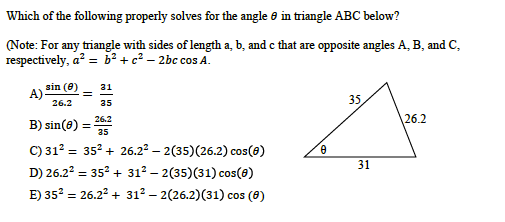Only D properly sets up the law of cosines with 26.2 on its own. It must be on its own since 26.2 is opposite of the angle in the given triangle.

Asymptotes

On the ACT, you will need to be familiar with how to find vertical and horizontal asymptotes.Asymptotes are lines that the graph never touches.

Vertical Asymptotes:

Vertical asymptotes occur when the denominator of a rational function is equal to zero. To find where the vertical asymptotes of a function are, you just need to set the denominator equal to zero and solve.Practice: Find the vertical asymptote(s) in the following function.Scroll down for the answer and solution.

Answer: The vertical asymptotes are at x = 0 and 3.Horizontal Asymptotes:

For horizontal asymptotes, you just need to find the highest power in the numerator and in the denominator and remember two simple rules:

(1) If the degree in the denominator is bigger than the degree in the numerator, than the horizontal asymptote is at y = 0.

As an example, let's find the horizontal asymptote for the following polynomial.Horizontal asymptote at y=0

In this polynomial, the degree in the denominator is 3 and the degree in the numerator is 2. Since the degree in the denominator (3) is greater than the degree in the numerator (2), the horizontal asymptote is a y=0.

(2) If the degree in the denominator is equal to the degree in the numerator, than the horizontal asymptote is found by dividing the leading coefficients of the polynomials.Since the degrees in the numerator and denominator are both 3, we must use the ratio of the coefficients to find what the horizontal asymptote will be.The horizontal asymptote is a y = 3

Practice: Find the vertical and horizontal asymptotes for the following functions.Scroll down for the answers and solutions.

1) Horizontal asymptote at y = 0, vertical asymptotes at x = -1, 3

Since the degree of the denominator (2) is greater than the degree in the numerator (1), the horizontal asymptote is at y = 0.

To find the vertical asymptotes, set the denominator equal to zero and solve.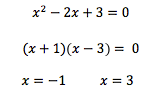2) Horizontal asymptote at y = 5, vertical asymptotes at x = 0

Since the degree of the denominator (3) and numerator (3) are the same, we use the ratio of the coefficients to find the horizontal asymptote. 5/1 = 5, so the horizontal asymptotes is at y = 5.

To find the vertical asymptote, we set the denominator equal to zero.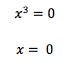Complex Numbers and the Complex Number Plane

Complex Numbers:

Complex numbers have an imaginary portion and a real portion. Remember that if you have a graphing calculator, you can simply type in most complex number problems into your calculator using the "i" button and let your calculator solve them problem for you.

In case you do not have a graphing calculator, here's what you need to know:Good thing complex number are not nearly this complex!

Complex Number Plane:

The complex number plane is very similar to the regular coordinate plane. The x-axis is used for the real number part of a complex number while the y-axis is used for the imaginary number part.

For any complex number a + bi, plot it as point (a,b) on the complex number plane.

As an example, let's see where 2 + 3i appear on the complex number plane:As long as you remember that the real number is on the x-axis and the imaginary number is on the y-axis, math problems on this topic will be easy.

Absolute Value of Complex Numbers:

The absolute value of a complex number is the distance from zero (the origin) to that number on the complex number plane.This formula may look complex, but it is just the pythagorean theorem.

For example, let's see how to find the absolute value of 3 + 4iIt's that easy!

Practice: Find the absolute values of the following imaginary numbers.

1) 7 - 5i

2) 12 + 5i

3) -6 - iDistance Between Complex Numbers in the Complex Plane:

To find the distance between complex numbers, you just need to use the distance formula.Here's an example so you can see how it works just like the normal distance formula.

Find the distance between, in coordinate units, between the points -2 + 4i and 1 - 3i on the complex plane.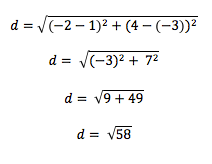Practice: Find the distance between the following pairs of complex numbers on the complex plane.

1) 9 + 2i and 7 - 4i

2) -5 + i and 1 + 5i

Scroll down for the answers and solutions.